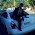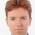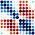## Friday, March 13, 2009

### Visulization of correlation matrix

• Color Image
`data(mtcars)fit = lm(mpg ~ ., mtcars)cor = summary(fit, correlation = TRUE)\$correlationcor2 = t(cor[11:1, ])colors = c("#A50F15", "#DE2D26", "#FB6A4A", "#FCAE91", "#FEE5D9","white", "#EFF3FF", "#BDD7E7", "#6BAED6", "#3182BD", "#08519C")image(1:11, 1:11, cor2, axes = FALSE, ann = F, col = colors)text(rep(1:11, 11), rep(1:11, each = 11), round(100 * cor2))`• Ellipses
`library(ellipse)col = colors[as.vector(apply(corr, 2, rank))]plotcorr(cor, col = col, mar = rep(0, 4))`
`circle.cor = function(cor, axes = FALSE, xlab = "",     ylab = "", asp = 1, title = "Taiyun's cor-matrix circles",     ...) { n = nrow(cor) par(mar = c(0, 0, 2, 0), bg = "white") plot(c(0, n + 0.8), c(0, n + 0.8), axes = axes, xlab = "",         ylab = "", asp = 1, type = "n") ##add grid segments(rep(0.5, n + 1), 0.5 + 0:n, rep(n + 0.5, n + 1),         0.5 + 0:n, col = "gray") segments(0.5 + 0:n, rep(0.5, n + 1), 0.5 + 0:n, rep(n + 0.5,                 n), col = "gray") ##define circles' background color. ##black for positive correlation coefficient and white for negative bg = cor bg[cor > 0] = "black" bg[cor <= 0] = "white"   ##plot n*n circles using vector language, suggested by Yihui Xie  symbols(rep(1:n, each = n), rep(n:1, n), add = TRUE, inches = F,    circles = as.vector(sqrt(abs(cor))/2), bg = as.vector(bg))  text(rep(0, n), 1:n, n:1, col = "red")  text(1:n, rep(n + 1), 1:n, col = "red")  title(title) } ## an example data(mtcars) fit = lm(mpg ~ ., mtcars) cor = summary(fit, correlation = TRUE)\$correlation circle.cor(cor) `The circles with black background denote positive correlation coefficient, and the area of circles denotes the absolute value. See more in my Picasa here.

The above three graphs based on the same data. Dear friends, which gives your more information at first galance?

#### 18 comments:

1.Very nicely done! Good to see you are an R user as well. I wonder if you perhaps saw my post on optimising R here?

2.Thanks for the compliment:)
Yes, I happened to see your blog last night, and I really learned a lot from it:)

3.What I like most about your circle plot for the correlation matrix is its simplicity. Besides, I think you can generalize this plot to the distance matrix, which can be used to demonstrate cluster analysis. Correlation is also a kind of distance.

4.Good work. The graph is now on the graph gallery

One possible enhancement would be to somehow illustrate that the the correlation is significantly different to zero (cor.test). Although this would need to take the data as input and not just the correlation matrix.

5.I'll add to the complements - I've been wondering about good graphical presentations of correlation matrices, but this is the best I've seen.

One suggested, - at Revolutions they pointed out that the white circles disappear on the white background. Could you simply change the background to "grey50"? I tried it last night and I (at least) like it.

6.to Bob O'Hara:Thanks for the comment on my blog. I appreciate your wonderful idea! And now I put some different graph of correlation matrix circles on my Picasa:http://picasaweb.google.com/WeiTaiyun/CorrelationMatrixCircles#
Welcome any comment.

7.I like the last of the three plots---it's simple yet elegant and most suitable for print (b&w).

8.Dear Taiyun,

Nice correlation plots on your Picasa. Do you have the R code for generating these?

Thanks,
Ravi.

9.To Ravi:
Yes, I have. But I don't know your email and I can't see your website, my email is weitaiyun[at]gmail.com

10.Hi - It is a well known psychophysical phenomenon that humans cannot perceive area very accurately. You are better off using luminance or hue.

11.Hi Taiyun,

Thanks for creating this blog... it gives a vivid pictorial depiction of the correlation matrix.
Thanks also for sending me the image corresponding to the Boston Housing data set. I plan to use it for an upcoming presentation.

12.Good to see you are an R user as well

Work from home India

13.I like the last one, I am trying to use it with my data because I have over 60 variables, but I am getting stuck somewhere... Could you help me out jorge.eco.ramos at gmail dot com ?

14.Pretty nice article on visualizing correlation matrices. Thanks for contributing.

15.You leave so useful information,I will share this info with my friends.
Louis Vuitton France
Louis Vuitton Paris
Louis Vuitton Speedy
Louis Vuitton Sac Prix

16.Thanks! How about having an option allowing to display a correlation matrix similar to http://www.mathworks.com/help/econ/corrplot.html , which shows both the correlation coefficient and and the correlation plot for each cell of the correlation matrix?

1.library(corrplot)
?corrplot

Notice addCoef.col

17.